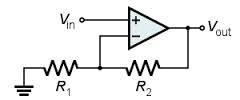## Op Amp Bandwidth

Enter the following: R1, K, fu and select Config. Then click on "Get_R2, Kn and fc".

1. The Bandwidth (fc) is sinply the Unity-Gain Frequency (fu)
reduced by the Noise Gain (Kn):
fc = fu / Kn
where Kn = (R1+R2)/R1 for either config
2. For the same gain, you get more bandwidth from the Non-Inverting amplifier.
Compare bandwidths for K=1,2,... for both configs..
The effect is less pronounced at higher gains.

 SchematicConfig Non-Inverting Inverting

 R1 (ohms) Gain Resistor 1 K=Vout/Vin Knon = (R1+R2)/R1, Kinv = -R2/R1 fu (Hz) Unity Gain Bandwidth of Op Amp R2 (Ohms) R2non = R1*(K-1), R2inv = R1*K Kn (V/V) Noise Gain K = (R1+R2)/R1 fc (Hz) Bandwidth fc=fu/Kn

To run or modify on your PC, download a simple version of this file with image (*.zip).
For a quick tour of some JavaScript / HTML basics, check out Inside the Code.

### JavaScript

This code features one function call associated with the "Get_R2, Kn and fc" button. Here's the code.

```//////////////////////////////////////////////
// calc BW
//////////////////////////////////////////////
function get_OpAmpBW() {
// get values directly from form
var R1=document.myForm.R1.value;
var K=document.myForm.K.value;
var fu=document.myForm.fu.value;
// convert text to number
R1=Number(R1);
K=Number(K);
fu=Number(fu);

var R2, Kn, fc;

// take absolute value of gain
K = Math.abs(K);

// calc R2
// non-invert - config or invert - config
if (document.myForm.config.checked) R2=R1*(K-1);
if (document.myForm.config.checked) R2=R1*K;

// calc noise gain and bandwidth
Kn = R2/R1+1;
fc = fu / Kn;

// place in text box
document.myForm.R2.value = (R2).toPrecision(2);
document.myForm.Kn.value = (Kn).toPrecision(2);
document.myForm.fc.value = (fc).toPrecision(2);
}
```

The two radio buttons, both named "config", create an array of elements config and config for the buttons named "Non-Inverting" and "Inverting". You can use the array to determine which button was checked as shown in the code above.

### HTML

Here's the HTML for the radio butons to select the amplifier configuration. Note that both are named "config". You can set which button is initially selected by including "checked" in the input line.